# Simplifying Expressions with Rational Exponents

An error occurred trying to load this video.

Try refreshing the page, or contact customer support.

Coming up next: How to Graph Cubics, Quartics, Quintics and Beyond

### You're on a roll. Keep up the good work!

Replay
Your next lesson will play in 10 seconds
• 0:05 Expressions with…
• 0:41 Examples 1-4
• 2:19 Examples 5-7
• 6:07 Radical to Rational…
• 6:27 Example 8
• 7:17 Lesson Summary

Want to watch this again later?

Timeline
Autoplay
Autoplay
Speed

#### Recommended Lessons and Courses for You

Lesson Transcript
Instructor: Kathryn Maloney

Kathryn teaches college math. She holds a master's degree in Learning and Technology.

Simplifying expressions with rational exponents is so easy. In fact, you already know how to do it! We simply use the exponent properties but with fractions as the exponent!

## Expressions with Rational Exponents

Rational exponents follow exponent properties except using fractions.

Review of exponent properties - you need to memorize these. Just can't seem to memorize them? Have you tried flashcards? They work fantastic, and you can even use them anywhere!

1. Product of Powers: xa*xb = x(a + b)
2. Power to a Power: (xa)b = x(a * b)
3. Quotient of Powers: (xa)/(xb) = x(a - b)
4. Power of a Product: (xy)a = xaya
5. Power of a Quotient: (x/y)a = xa / ya
6. Negative Exponent: x(-a) = 1 / xa
7. Zero Exponent: x0 = 1

Putting the exponent rules to work with exponent properties...

## Example #1

y(1/2) * y(1/3)

For this one, we're going to follow the product of powers. Remember, when we multiply, we add their exponents.

1/2 + 1/3 = 5/6

So the answer is going to be y(5/6).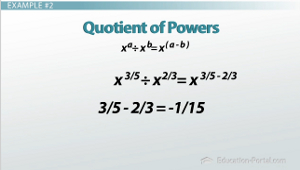## Example #2

Simplify: x(3/5) / x(2/3)

For this one, we're going to use the quotient of powers. Remember, when we divide, we subtract their exponents. So, we're going to have:

x(3/5 - 2/3)

3/5 - 2/3 = -1/15

So our answer is x(-1/15).

## Example #3

Simplify: x(-2/7)

For this one, we're going to use the negative exponents property. Remember, when we have a negative exponent, we flip it. If it's in the numerator, we flip it to the denominator, which is in this case.

So our answer is going to be 1 / (x(2/7)).

## Example #4

Simplify: (x(4/5))(3/4)

In this one, we have power to a power. We're going to have (x(4/5))(3/4), so we're going to multiply 4/5 * 3/4 which is 12/20. We need to reduce our fractions when we're going to get our final answer. 12/20 reduces to 3/5.

So our answer is going to be x(3/5).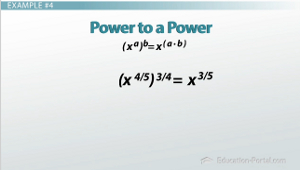## Example #5

Putting multiple exponent rules to work with exponent properties... Simplify using positive exponents. Always reduce the fractions to lowest terms.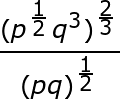First we're going to simplify the power to a power. So now we'll have: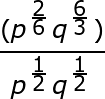Write like terms over each other, if necessary. Well, we already have the p's over the p's and the q's over the q's. There's no need to simplify fractions now. We're going to go right to simplifying quotient of powers. Remember, when we divide, we subtract their exponents. So we have:

p(2/6 - 1/2) * q(6/3 - 1/2)

That gives us:

p(-1/6) * q(9/6)

Next we need to reduce the fractions because we're almost to our answer. So we'll have:

p(-1/6) * q(3/2)

We want to rewrite these using positive exponents. Remember, if it's negative in the numerator, it flips to the denominator. So our final answer's going to be:

q(3/2) / p(1/6)

## Example #6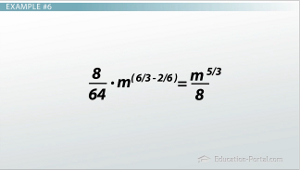Simplify using positive exponents. Always reduce the fractions to lowest terms. We're going to have: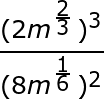We're going to simplify power to a power. So we'll have: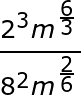Remember, power to a power means to multiply the exponents. Next, let's write like terms over each other. We already have 23 over 82 and m(6/3) over m(2/6). So let's move to the next step. There's no need to simplify fractions just yet, so we're going to simplify quotient of powers. Remember, when we divide, we subtract. So now we're going to have:

8/64 * m(6/3 - 2/6)

To unlock this lesson you must be a Study.com Member.

### Register to view this lesson

Are you a student or a teacher?

### Unlock Your Education

#### See for yourself why 30 million people use Study.com

##### Become a Study.com member and start learning now.
Back
What teachers are saying about Study.com

### Earning College Credit

Did you know… We have over 160 college courses that prepare you to earn credit by exam that is accepted by over 1,500 colleges and universities. You can test out of the first two years of college and save thousands off your degree. Anyone can earn credit-by-exam regardless of age or education level.